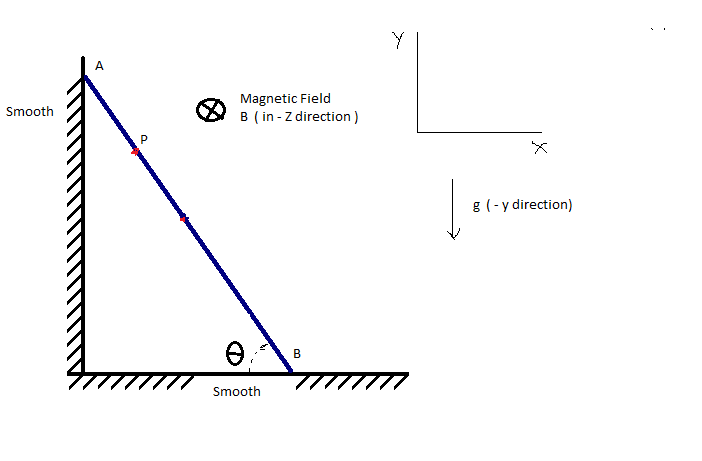# Mechanics + Magnetism = Mechatism 2Let an conducting Thin Rigid Rod of Length 'L' and mass 'm' is released under gravity from rest at time t=0 at an angular position ${ \theta }_{ 0 }\quad =\quad { 60 }^{ 0 }$ from horizontal.

There is gravity in -Y direction and uniform Magnetic Field of strength ${ B }_{ 0 }\quad$

The Rod is sliding on the perfectly smooth Surface.

So Find the Magnitude of Motional EMF produced in Rod between The End Point A and Point P at the instant when Rod is making an angle $\theta \quad =\quad { 30 }^{ 0 }$ from Horizontal.

Let your answer is $\alpha$ Then Evaluate $\left\lfloor 100\alpha \right\rfloor$.

Details

$\bullet \quad AP\quad =\quad \frac { 1 }{ 4 } \quad m\\ \bullet \quad L\quad \quad =\quad \quad 1\quad m\\ \\ \bullet \quad B\quad \quad =\quad \quad 1\quad T\\ \\ \bullet \quad g\quad \quad =\quad \quad 10\quad m/{ s }^{ 2 }$.

###### This is part of set Mixing of concept Click here

This is Original

×

Problem Loading...

Note Loading...

Set Loading...